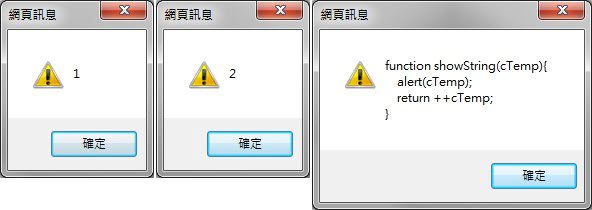﻿

# JavaScript Functions的Declarations與Expressions

## Function Declaration宣告式函式

``````showString("Hello world FRONT!");  //正確調用

function showString(cTemp){
}

showString("Hello world BEHIND!");  //正確調用
showString();  //正確調用但是會顯示空字串
showString;  //不會被調用執行（這個觀念很簡單但很重要）
``````

``````function showString(cTemp){
return ++cTemp;
}
``````## Dynamic Function Declaration動態宣告式函式

``````/* Function Declaration寫成「在執行期建立」的方式 */

if(true){
function showString(cTemp){ alert("fn1:" + cTemp); }
}else{
function showString(cTemp){ alert("fn2:" + cTemp); }
}

//輸出 fn1:Hello world
showString("Hello world");

if(false){
function showString(cTemp){ alert("fn3:" + cTemp); }
}else{
function showString(cTemp){ alert("fn4:" + cTemp); }
}

//覆寫輸出 fn4:Hello world
showString("Hello world");
``````

## 將Function Declaration宣告式函式指派給一個變數

``````//一定可以調用成功
showString("Hello!");

//不可以運行，因為hi1是在下方執行期運行時才被指派的
//hi_1("Hello! with hi_1");

//將在執行前就被建置完成
function showString(cTemp){
if(cTemp === undefined)
return "foo";
else
}

//指派兩個變數，指向showString
var hi_1 = showString;
var hi_2 = showString();  //因為()而執行showString並傳入空參數，收到"foo"

hi_1("Hello! with hi_1");

``````

## Function Expression表達式函式

``````//Function Expression宣告（也就是匿名函式Anonymous Functions）
var callMe = function(cTemp){
if(cTemp === undefined)
return "Nothing";
else
}

callMe("TEST");  //傳回TEST
``````

## Dynamic Function Expression動態表達式函式

``````var callMe;

if(false){
callMe = function(cTemp){ alert("fn1:" + cTemp); }
}else{
callMe = function(cTemp){ alert("fn2:" + cTemp); }
}

callMe("Hello world"); //輸出fn2:Hello world
``````

## Function Expression真正的匿名化

``````//一般型Function Expression
var cal1 = function(a, b){ return a + b; }

//自我執行型Function Expression
var cal2 = function(a, b){ return a + b; }(2, 3);
``````

``````function(a, b){ return a + b; }(2, 3);
``````

``````(function(a, b){ return a + b; })(2, 3);  //這樣就合法了，但是沒有地方可以展示傳回值，你可以加個alert();來展示
``````

``````(function(){
/* some code... */
})();

//或者這樣也可以（就我大量看程式碼的經驗來看比較少人用）
(function(){
/* some code... */
}());
``````

## 利用Function Constructor來進行Expressions表達式函式的建立

``````var callMe = new Function("cTemp", "alert(cTemp);");  //記得F要大寫，它已經是class與instance的關係了
callMe("Hello, world!");
``````

## 利用Function Regular Value的特性來重構Expressions表達式函式

``````function wrapper(xCode){
}

//加法計算機，傳回300
wrapper(function(a, b){
return a + b;
});

//減法計算機，傳回-100
wrapper(function(a, b){
return a - b;
});
``````

``````function wrapper(xCode, x, y){
}

wrapper(function(a, b){
return a + b;
}, 100, 200);

wrapper(function(a, b){
return a - b;
}, 100, 200);
``````

## 具備名稱的Expressions表達式函式

``````var result = function Fibonacci(n){
return n === 1 ? n : n + Fibonacci(n - 1);
};